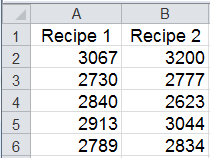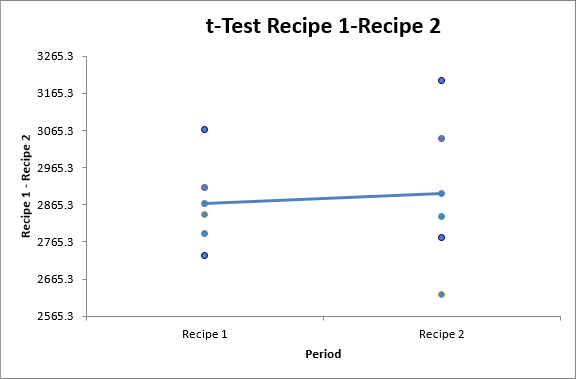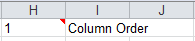# Want to Do a Two-Sample t-Test in Excel?

## QI Macros can do it for you!

### Create a t-Test in Excel using QI Macros:

2. Click on QI Macros menu > Statistical Tools > F & t Tests > t-Test Equal Variances.
3. QI Macros will do the math and analysis for you.

### You can do a t-Test in Excel using the Data Analysis Toolpak, but...

• Excel's interface can be confusing; QI Macros adds a new tab to Excel's menu making t-Tests point-and-click simple.
• Excel won't interpret your p-value for you; QI Macros Add-in will!
• Excel can't give you a Box and Whisker chart/Values Plot of the two factors; QI Macros Add-in can!

### Two-Sample t-Test Example

Lets say you're producing rubber made from two different recipes and you want to know if the tensile strengths are the same or different. You test five samples of each to get the data:#### Next Determine if the Variances are Equal or Unequal

In order to select the correct Two Sample t-Test, you need to determine if the variances are equal or unequal. QI Macros offers two options:

• Use the QI Macros Stat Wizard. It will run an F-test and then automatically choose the right t-Test for you.
• Run an F-test on the data to determine if the variances are equal or unequal. Then run the appropriate t-Test.

#### To Run a t-Test Using QI Macros:

1. Select the data and click on QI Macros Menu > Statistical Tools > F & t Tests > t-Test: Two-Sample Assuming Equal Variances or Unequal Variances, depending on the F-test results:
2.3. QI Macros will prompt you for a significance level:4. Note: Significance Level = (1 - Confidence Level).

5. Next, QI Macros will prompt for the hypothesized difference in the means (default is 0).6. QI Macros will perform the calculations and interpret the results for you:
7.8. In the July 2022 version of QI Macros, the Box & Whisker Plot output was removed and replaced with a Values Plot output, as Values Plots provide simpler visual representations of your data.:
9.QI Macros compares the p-value (0.818) to the significance level (0.05) and tells you "Cannot Reject the Null Hypothesis/Accept the Null Hypothesis, because p>0.05 (Means are not Different/Means are the Same)."

QI Macros also draws a Box Plot to help you visualize the difference between the variances (height of box and length of whiskers) and means (line of box where the color changes).

Tip: If you specify a mean difference other than zero, use:
• two-tail p value (Difference = Hypothesized Mean Difference)
• one-tail p value (Difference < Hypothesized Mean Difference)
• 1-one tail p value (Difference > Hypothesized Mean Difference)

NOTE: If your t-Test determines Means are not Different, than your TOST output will state "Cannot conclude means are equivalent."

#### To change the order of your Two-Sample t-Test results between the first and last columns:

2. Then, in row 1 you have the option to update the "Column Order" by inputting value "1" (Column 1/Column 2) or "2" (Column 2/Column 1):
3.NOTE: Changing the order of your t-Test results will not change the order of the Box and Whisker output.

### Why Choose QI Macros Statistical Software for Excel?### Easy to Use

• Works Right in Excel
• Interprets p-values for You
• Accurate No-Worry Results
• Free Training Anytime### Proven and Trusted

• 100,000 Users in 80 Countries
• Celebrating 20th Anniversary
• Five Star CNET Rating - Virus Free### Affordable

• Only \$349 USD
Quantity Discounts Available
• No annual fees
• Free Technical Support